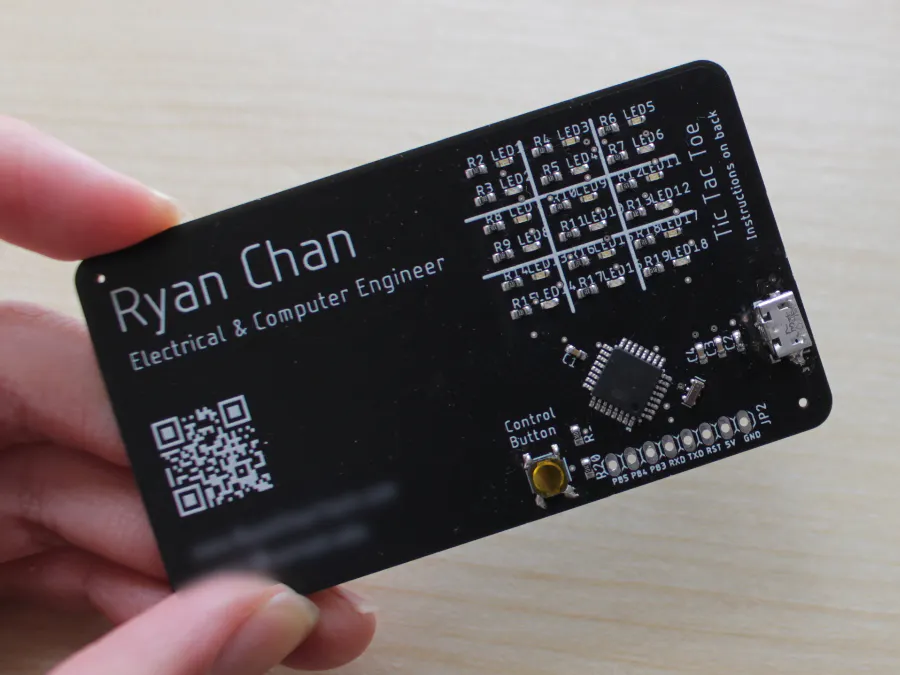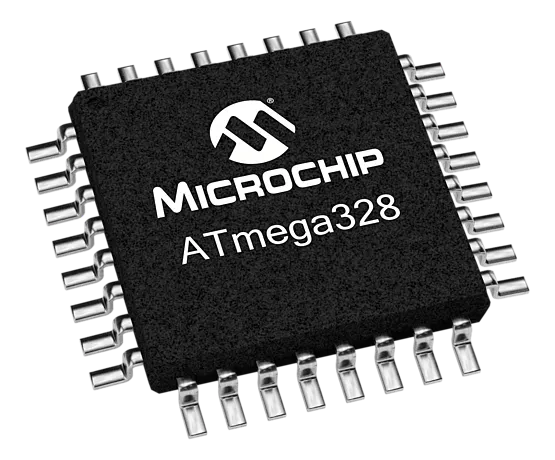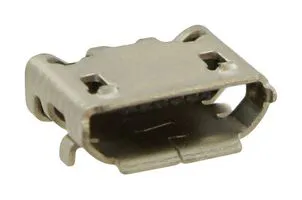# Tic Tac Toe on a Business Card

A business card that can play the classic game of Tic Tac Toe.## Things used in this project

### Hardware componentsMicrochip ATmega328
×1
 0603 SMD LED 9x Blue and 9x Red
×18
 0603 Resistors 2x 10k ohm, 19x 1k ohm
×21
 0603 Capacitors 1x 10uF, 2x 0.1uF (for decoupling capacitors)
×3USB Connector, Micro USB Type B
×1
 SMD Button
×1
 16MHz SMD Ceramic Resonator
×1

### Software apps and online servicesArduino IDE
 Autodesk EAGLE

## Code

### Business Card Tic Tac Toe Code

Arduino
```int playerBlue = {{A0,0},{A2,0},{A4,0}, //{pin number, marked (0=empty, 1=marked)}
{0,0},{2,0},{4,0},
{6,0},{8,0},{10,0}};
int playerRed = {{A1,0},{A3,0},{A5,0}, //{pin number, marked (0=empty, 1=marked)}
{1,0},{3,0},{5,0},
{7,0},{9,0},{11,0}};

const int controlButtonPin = 13;

int currentPosition = 0;

int setPositions = 0; // used to see if the board is completely filled

short turn = 0; // 0 = blue's turn, 1 = red's turn

boolean singlePlayer = false;

void setup() {
pinMode(0,OUTPUT);
pinMode(1,OUTPUT);
}

void loop() {
checkWinner();

int buttonHoldTime = 0;

buttonHoldTime++;
delay(1);
}

if(buttonHoldTime > 1 && buttonHoldTime < 250){
nextPosition();
}else if(buttonHoldTime >= 250 && buttonHoldTime <= 5000){
setPositions ++;
setPosition();
nextPosition();

if(singlePlayer){
computerMove();
}
}else if(buttonHoldTime > 5000){
singlePlayer = !singlePlayer;
resetGame();
displayMode();
}else{
displaySetPositions();
}
}

void nextPosition(){
checkWinner();

boolean positionDecided = false;

while(!positionDecided){
currentPosition ++;
if(currentPosition > 8){
currentPosition = 0;
}

if(playerBlue[currentPosition] == 0 && playerRed[currentPosition] == 0){
positionDecided = true;
}
}
}

void setPosition(){
if(turn == 0){
playerBlue[currentPosition] = 1;
turn = 1;
}else{
playerRed[currentPosition] = 1;
turn = 0;
}
}

if(turn == 0){ //when it's blue's turn
digitalWrite(playerBlue[currentPosition], HIGH);
delay(100);
digitalWrite(playerBlue[currentPosition], LOW);
delay(100);
}else{
digitalWrite(playerRed[currentPosition], HIGH);
delay(100);
digitalWrite(playerRed[currentPosition], LOW);
delay(100);
}
}

void displaySetPositions(){
for(int i = 0; i < 9; i++){
digitalWrite(playerBlue[i],playerBlue[i]);
digitalWrite(playerRed[i],playerRed[i]);
}
}

void resetGame(){
for(int i = 0; i < 9; i++){
playerBlue[i] = 0;
playerRed[i] = 0;
}

currentPosition = 0;
setPositions = 0;
turn = 0;
}

void displayMode(){
if(singlePlayer){
PORTB = B00000011;
PORTC = B00001100;
PORTD = B00001100;
}else{
PORTB = B00001011;
PORTC = B00110100;
PORTD = B00011100;
}
delay(2000);

PORTB = B00000000;
PORTC = B00000000;
PORTD = B00000000;
}

void computerMove(){
for(int i = 0; i < random(9); i++){
nextPosition();
}
setPosition();
nextPosition();
}

void checkWinner(){
boolean blue = false;
boolean red = false;
boolean gameEnd = false;

// this is VERY sloppy. I plan to make a better algorithm soon
if((playerBlue == 1 && playerBlue == 1 && playerBlue == 1) ||
(playerBlue == 1 && playerBlue == 1 && playerBlue == 1) ||
(playerBlue == 1 && playerBlue == 1 && playerBlue == 1) ||
(playerBlue == 1 && playerBlue == 1 && playerBlue == 1) ||
(playerBlue == 1 && playerBlue == 1 && playerBlue == 1) ||
(playerBlue == 1 && playerBlue == 1 && playerBlue == 1) ||
(playerBlue == 1 && playerBlue == 1 && playerBlue == 1) ||
(playerBlue == 1 && playerBlue == 1 && playerBlue == 1))
{
blue = true;
gameEnd = true;
}
else if((playerRed == 1 && playerRed == 1 && playerRed == 1) ||
(playerRed == 1 && playerRed == 1 && playerRed == 1) ||
(playerRed == 1 && playerRed == 1 && playerRed == 1) ||
(playerRed == 1 && playerRed == 1 && playerRed == 1) ||
(playerRed == 1 && playerRed == 1 && playerRed == 1) ||
(playerRed == 1 && playerRed == 1 && playerRed == 1) ||
(playerRed == 1 && playerRed == 1 && playerRed == 1) ||
(playerRed == 1 && playerRed == 1 && playerRed == 1))
{
red = true;
gameEnd = true;
}

if(setPositions > 8){
blue = true;
red = true;
gameEnd = true;
}

if(gameEnd){
for(int j = 0; j < 5; j++){
for(int i = 0; i < 9; i++){
digitalWrite(playerBlue[i],blue);
digitalWrite(playerRed[i],red);
}
delay(500);

for(int i = 0; i < 9; i++){
digitalWrite(playerBlue[i],LOW);
digitalWrite(playerRed[i],LOW);
}
delay(500);
}
resetGame();
}
}
```

## Credits

### Ryan Chan

9 projects • 222 followers
I like turtles. I also like robots.